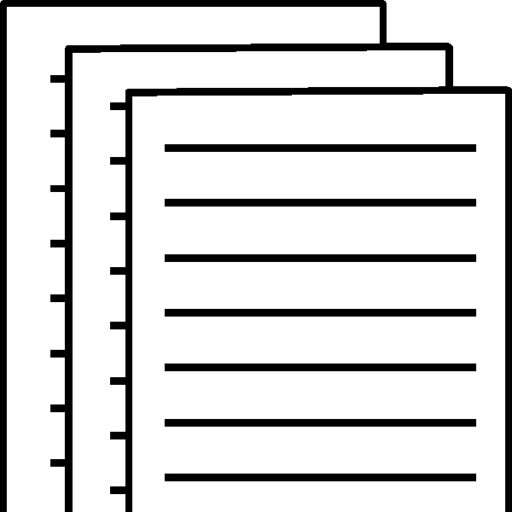## math 215 ch2b problem-solving with units-1

Approaches to Problem Solving

2

Unit 2B

Problem-Solving with Units

Unit Analysis in Problem Solving

Step 1. Identify the units involved in the problem and the units that you expect for the answer.Step 2. Use the given units and the expected answer units to help you find a strategy for solving the problem. Be sure to perform all operations (such as multiplication or division) on both the numbers and their associated units.

style.visibility

Unit Analysis in Problem Solving

Remember:• You cannot add or subtract numbers with different units, but you can combine different units through multiplication, division, or raising to powers.• It is easier to keep track of units if you replace division with multiplication by the reciprocal. For example, instead of dividing by 60 s/min, multiply by 1 min/60 s.Step 3. When you complete your calculations, make sure that your answer has the units you expected. If it doesn’t, then you’ve done something wrong.

style.visibilitystyle.visibility

Example

You are buying 30 acres of farm land at \$12,000 per acre. What is the total cost?SolutionStep 1. The question asks about total cost, and one of the given units is dollars, so we expect an answer in dollars.Step 2. The key word of suggests multiplication, and we realize that we can end up with an answer in dollars by multiplying the acreage by the cost per acre:

style.visibilitystyle.visibilitystyle.visibility

Example

You are buying 30 acres of farm land at \$12,000 per acre. What is the total cost?Step 3. We have found that the land costs \$360,000. Note that the answer has come out with the units we expect.

style.visibility

Example

You are planning to make pesto and need to buy basil. At the grocery store, you can buy small containers of basil priced at \$2.99 for each 2/3-ounce container. At the farmer’s market, you can buy basil in bunches for \$12 per pound. Which is the better deal?SolutionTo compare the prices, we need them both in the same units. Convert the small container price to a price per pound.

style.visibilitystyle.visibilitystyle.visibility

Step 1 . We have to convert everything to the same units i.e. price per poundStep 2 .The keyword is Multiplication . We already have Farmer’s market \$12 per poundWe are to convert \$2.99 per 2/3-ounce container to Dollars per pound. 1 pound = 16 ounces((2/3 ounce) /16 ounce ) ×1Pund= 2/(3×16)= 2/48= 1/24\$2.99 per 1/24 pound Converting to 1 pound \$2.99 × 24 = 71.76\$71.76 per pound

Solution

Step 3 .Farmer’s market – \$12 per poundVs Small Containers – \$71.76 per pound From the calculations, it is clear that the small container is more expensive hence the farmer’s market is a better deal. Note, the answer is in the units we expected.

Solution

Density describes compactness or crowding.

Material density is given in units of mass per unit volume.e.g., grams per cubic centimeter (g/cm3)Population density is given by the number of people per unit area.e.g., people per square mile (people/mi2)Information density is given in units of mass per unit volume.e.g., gigabytes per square inch (GB/in.2)

Units of Density and Concentration

style.visibilityppt_xppt_ystyle.visibilityppt_xppt_ystyle.visibilityppt_xppt_ystyle.visibilityppt_xppt_ystyle.visibilityppt_xppt_ystyle.visibilityppt_xppt_ystyle.visibilityppt_xppt_y

The concentration of an air pollutant is often measured by the number of molecules of the pollutant per million molecules of air.e.g., parts per million (ppm)Blood alcohol content (BAC) describes the concentration of alcohol in a person’s body.e.g., grams of alcohol per 100 milliliters of blood

Concentration describes the amount of one substance mixed with another.

Units of Density and Concentration

style.visibilityppt_xppt_ystyle.visibilityppt_xppt_ystyle.visibilityppt_xppt_ystyle.visibilityppt_xppt_ystyle.visibilityppt_xppt_y

Example

A child weighing 15 kilograms has a bacterial ear infection. A physician orders treatment with amoxicillin at a dosage based on 30 milligrams per kilogram of body weight per day, divided into doses every 12 hours. a. How much amoxicillin should the child be prescribed every 12 hours?b. If the medicine is to be taken in a liquid suspension with concentration 25 mg/ml, how much should the child take every 12 hours?

a) Step 1 . The answer should be in milligrams Step 2 . The key to this is multiplication(15 kg×30mg)/1kg = 450 milligrams Step 3 . The answer is in milligramsb)Step 1) we should have the answer in milliliters that’s the available concentration. Step 2 ) The key to this is division (450mg ÷ 25mg) × 1 ml = 18 mlStep 3) . The answer to this is 18 ml, the units suspension available is milligrams

Solution

To view and download a complete answer, scroll down to the bottom to pay